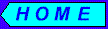# Portfolio Analysis

A portfolio is a collection of securities owned by an investor. The calculation of expected return of portfolio is straightforward, but calculation of variance and standard deviation involves covariance and correlation, statistical measures that quantify the relationship between the returns of two different securities.

## Expected Return of Portfolio

Since each security’s future return may be considered as a random variable, the return of a portfolio also can be thought as a random variable dependant on expected returns of the individual securities that make up the portfolio. The expected return of portfolio is a weighted sum of the expected returns of the individual securities:

Portfolio Expected Return = A*r(a) + B*r(b) + C*P(c) +... ,

where A, B, C, ... are relative weights of securities a, b, c, ... in portfolio and r(a), r(b), r(c) , ... are corresponding expected returns of securities. Relative weight of each security in portfolio is a fraction of this security’s present value in the total present value of the portfolio:

Weight of Security(x) = val(x) /(Total Value of Portfolio),

where "val" means present value.

Let’s consider numeric example. First we compute relative weights of securities in portfolio:

```	Security:	Present Value:		Relative Weight:
a		     \$400		\$400/\$2500 = 0.16
b		     \$900		\$900/\$2500 = 0.36
c		     \$700		\$700/\$2500 = 0.28
d		     \$500		\$500/\$2500 = 0.20
---------------------------------------------------------
Total:		    \$2500			      1.0
```

Now we can calculate expected return of portfolio R:

```	Security:    Weight:    Expected Ret:
a	      0.16	     5%		 0.16 *   5% = 0.80%
b	      0.36	     3%		 0.36 *   3% = 1.08%
c	      0.28	     7%		 0.28 *   7% = 1.96%
d	      0.20	    11%		 0.20 *  11% = 2.20%
------------------------------------------------------------
Portfolio Expected Return = 6.04%
```

## Covariance and Correlation

The portfolio variance and standard deviation are used as a measures to quantify the portfolio risk. For their calculation we need to compute covariance and correlation between securities of portfolio. Covariance is a statistical quantity which measures the relationship between two random variables. In case of returns of financial securities covariance measures relationship between returns of two securities:

Covariance(a,b) = Expected Value of [( A-r(a) )*( B-r(b) )],

where A and B are returns and r(a) and r(b) are expected values of a and b securities. If random variables A and B are discrete (i.e. their values can be listed), the above formula will take the form:

```			Covariance(a,b) = [x-r(a)]*[y-r(b)]*P(x,y) + [y-r(a)]*[z-r(b)]*P(y,z) +
+ [x-r(a)]*[z-r(b)]*P(x,z) + ...
```
where x, y, z, ... are particular returns for securities a and b, P(x,y) is the joint probability of x and y, etc. A large positive covariance implies that the returns move together, i.e. if one security’s return is high then other security’s return is likely to be high. Large negative covariance signifies the opposite trend in movements of returns of two securities, i.e. if one security’s return is high, the other security’s return is likely to be low. Finally, if covariance is close to zero then two securities have very weak relationship, or in other words, movements of returns of two securities are not related to each other. But what does large mean, what is the comparison criteria? Given the computed value of covariance, it’s difficult to say whether it is large or small. This is where the correlation is useful. The correlation is computed by dividing the covariance by the standard deviations of the two securities:

Correlation(a,b) = Covariance(a,b) / ( St.Dev.(a)* St.Dev.(b) )

Since standard deviations are positive values, the correlation always has the same sign as covariance. The division of covariance by standard deviations forces values of correlation to be between -1 and 1. -1 means perfect negative relationship between securities, 1 means perfect positive relationship, and values close to zero means weak relationship between movements of returns of two securities.

## Variance and Standard Deviation of Portfolio

Now we can compute variance and standard deviation of portfolio:

Variance of Portfolio = A*B*cv(a,b)+B*C*cv(b,c)+A*C*cv(a,c)+A*D* cv(a,d) + ... ,

where A, B, C, D, ... are corresponding relative weights of a,b,c,d, ... securities in portfolio and "cv()" symbol means covariance. Standard deviation of portfolio is a square root from variance value:

Standard Deviation of Portfolio = sqrt( Variance of Portfolio ),

where "sqrt" means square root. For simplest case of the portfolio consisting of two securities the variance will be:

Variance(a,b) = A*A * cv(a,a) + A*B*cv(a,b)+B*A*cv(b,a)+B*B*cv(b,b)

The covariance of security a with itself cv(a,a) equals to variance of the security, and similarly cv(b,b) equals to variance of security b. Then above formula for portfolio variance takes the form:

Variance(a,b) = sq(A)*var(a) + sq(B)*var(b) + 2*A*B*cv(a,b),

Where "sq" means square, "var" means, variance, "cv" means covariance, A and B are relative weights of securities a an b in portfolio.Customer Service: gsharia@yahoo.com